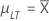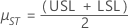# Calculations for process statistics and capability values for Process Report

Minitab calculates process statistics and capability values, as shown below.

## Unbiasing constants for estimating long-term and short-term standard deviation

Unbiasing constant for estimating long-term standard deviation
Unbiasing constant for estimating short-term standard deviation

where:

### Notation

TermDescription
c4(LT)jUnbiasing constant for long-term calculations at cumulative jth subgroup
c4(ST)jUnbiasing constant for short-term calculations at cumulative jth subgroup
df(LT)j Long-term degrees of freedom at jth subgroup
df(ST)j Short-term degrees of freedom at jth subgroup

## Short-term standard deviations

Short-term standard deviation with unbiasing constant (default)
Short-term standard deviation without unbiasing constant

where:

### Notation

TermDescription
Cum SD(ST)j Cumulative short-term standard deviation up to the jth subgroup
c4(ST)jUnbiasing constant for short-term calculations at cumulative jth subgroup
df(ST)j Short-term degrees of freedom at jth subgroup

## Long-term standard deviations

Long-term standard deviation without unbiasing constant (default)
Long-term standard deviation with unbiasing constant

where:

### Notation

TermDescription
Cum SD(LT)j Cumulative long-term standard deviation up to the jth subgroup
c4(LT)j Unbiasing constant for long-term calculations at cumulative jth subgroup
df(LT)j Long-term degrees of freedom at jth subgroup

## Long-term process mean

### Notation

TermDescription
µLT

Long-term mean or process mean

μLT = LT,K

###### Note## Long-term process standard deviation

σLT = Cum SD(LT)K

## Short-term process mean

If target is given
μST = T
If both specs are given (no target)If one spec is given (no target)
μST = μLT

### Notation

TermDescription
µST Short-term mean
TTarget
µLT

Long-term mean or process mean

###### Note## Capability statistics

CCpk
Cp
Cpk
CPL
CPU
###### Note

Cp, Cpk, and CCpk represent the potential capability of the process. Therefore, these formulas use short-term variability.

Pp
Ppk
PPL
PPU
###### Note

Pp and Ppk represent the actual process performance. Therefore, these formulas use long-term variability.

## Degrees of freedom

### Notation

TermDescription
df(LT)j Long-term degrees of freedom at jth subgroup
df(ST)j Short-term degrees of freedom at jth subgroup

## Probabilities

P.LSL(LT)j

Long-term probability of less than or equal to lower spec at jth subgroup

P.LSL(LT) j = 1 – Φ(Z.LSL(LT)j)

P.LSL(ST) j

Short-term probability of less than or equal to lower spec at jth subgroup

P.LSL(ST) j = 1 – Φ(Z.LSL(ST)j)

P.USL(LT) j

Long-term probability of greater than or equal to upper spec at jth subgroup

P.USL(LT) j = 1 – Φ(Z.USL(LT)j)

P.USL(ST) j

Short-term probability of greater than or equal to upper spec at jth subgroup

P.USL(ST) j = 1 – Φ(Z.LSL(ST)j)

P.Total(LT) j

Total (long-term) probability of out-of-spec at jth subgroup

P.Total(LT) j = P.USL(LT)j + P.LSL(LT)j

P.Total(ST)j

Total (short-term) probability of out-of-spec at jthsubgroup

P.Total(ST)j = P.USL(ST)j + P.LSL(ST)j

## Z.bench statistics

Z.Bench(LT)j

Benchmark Z (long-term) at jth subgroup

Z.Bench(LT)j = Φ−1(P.Total(LT)j)

Z.Bench(ST)j

Benchmark Z (short-term) at jth subgroup

Z.Bench(ST)j = Φ−1(P.Total(ST)j)

Z.LSL(LT)j

Z-value (long-term) for lower spec at jth subgroup

Z.LSL(LT)j = (μLT – LSL) / Cum SD(LT)j

Z.LSL(ST)j

Z-value (short-term) for lower spec at jth subgroup

Z.LSL(ST)j = (μST – LSL) / Cum SD(ST)j

Z.USL(LT)j

Z-value (long-term) for upper spec at jth subgroup

Z.USL(LT)j = (USL – μLT) / Cum SD(LT)j

Z.USL(ST)j

Z-value (short-term) for upper spec at jth subgroup

Z.USL(ST)j = (USL – μST) / Cum SD(ST)j

Z.Shiftj

Shift factor at jth subgroup

Z.Shiftj = Z.Bench(ST)j – Z.Bench(LT)j

By using this site you agree to the use of cookies for analytics and personalized content.  Read our policy